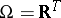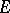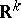# Algebra of sets

(diff) ← Older revision | Latest revision (diff) | Newer revision → (diff)

A non-empty collection of subsets of some setthat is closed under the set-theoretic operations (of union, intersection, taking complements), carried out a finite number of times. In order for a class of subsets of a setto be an algebra of sets, it is necessary (and sufficient) for it to be closed under finite unions and taking the complement. An algebra of sets that is closed under countable unions is known as a-algebra of sets. Any-algebra of sets is closed under the set-theoretic operations carried out a countable number of times.

### Examples.

1) The collection of finite subsets of an arbitrary setand their complements is an algebra of sets; the collection consisting of the at most countable subsets ofand their complements is a-algebra of sets.

2) The collection of finite unions of intervals of the typeforms an algebra of sets.

3) letbe a topological space; the-algebraof sets generated by the open subsets of(in other words, the smallest-algebra of sets containing all open subsets of) is known as the Borel-algebra of subsets of, while the sets belonging toare known as Borel sets.

4) let, whereis an arbitrary set (i.e.is the set of all real functions on); the classof sets of the typewhereis a Borel subset of, is an algebra of sets; in the theory of random processes a probability measure is often originally defined only on an algebra of this type, and is their subsequently extended to a wider class of sets (to the-algebra generated by).

5) The collection of Lebesgue-measurable subsets ofis a-algebra of sets.

Algebras (respectively,-algebras) are the natural domain of definition of finitely-additive (respectively,-additive) measures. According to the theorem of extension of measures, any-finite,-additive measure, defined on an algebra, can be uniquely extended to a-additive measure defined on the-algebra generated by.

How to Cite This Entry:
Algebra of sets. Encyclopedia of Mathematics. URL: http://encyclopediaofmath.org/index.php?title=Algebra_of_sets&oldid=15704
This article was adapted from an original article by V.V. Sazonov (originator), which appeared in Encyclopedia of Mathematics - ISBN 1402006098. See original article